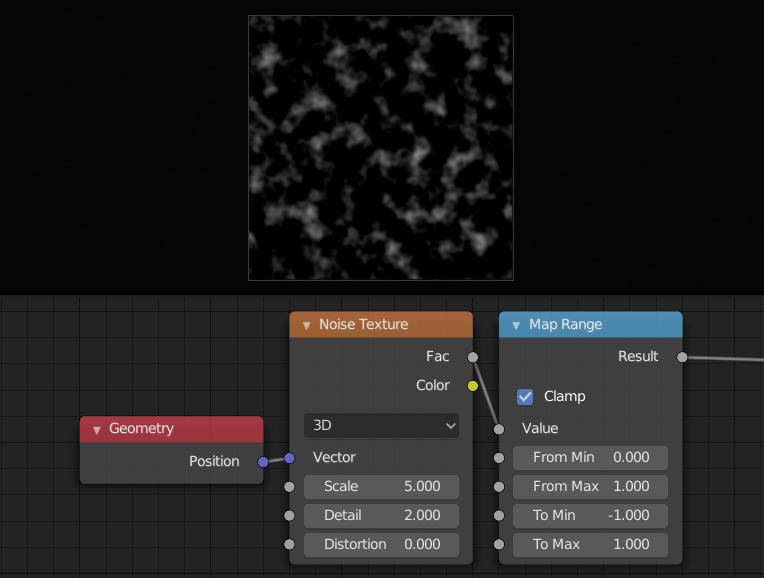# Map Range Node¶Map Range Node.

The Map Range node remaps a value from a range to a target range.

Value

The input value to be remapped.

From Min

The lower bound of the range to remap from.

From Max

The higher bound of the range to remap from.

To Min

The lower bound of the target range.

To Max

The higher bound of the target range.

Steps

The number of values allowed between To Min and To Max when using Stepped Linear interpolation. A higher value will give a smoother interpolation while lower values will progressively quantize the input.

## Properties¶

Interpolation Type

The mathematical method used to transition between gaps in the numerical inputs.

Linear (Lineal)

Linear interpolation between From Min and From Max values.

Stepped Linear

Stepped linear interpolation between From Min and From Max values.

Smooth Step

Smooth Hermite edge interpolation between From Min and From Max values.

Smoother Step

Smoother Hermite edge interpolation between From Min and From Max values.

Clamp

If enabled, the output is clamped to the target range.

## Salidas¶

Result

The input value after remapping.

## Ejemplos¶

The Noise Texture node outputs a value in the range [0, 1]. We can use the Map Range node to remap this value into the range [-1, 1].Example of Map Range node.